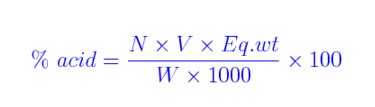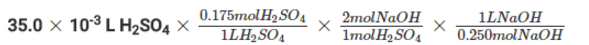# Titration Formula

A titration can define the volume of one solution required to react correctly with an identified volume of a different solution. Titration commonly comprises of reactions such as redox reactions and reactions involving precipitations, different than acid-base reactions.

“Titration can discern the volume of one solution required to react exactly with a known volume of a different solution.”

The equation for Titration Formula is articulated as:Where,

1000 = factor relating mg to grams

W = mass of sample

N = normality of titrant

V = volume of titrant

Eq.wt = equivalent weight of acid

Though commonly the Titration Formula is articulated as:Where,

V1 = Volume of titrant

N = Normality of titrant

V2 = Volume of sample

Eq.wt = equivalent weight of predominant acid

Solved Examples

Problem 1: Compute the titratable acidity if 17.5ml of 0.085N NaOH is required to titrate a 15ml sample of juice, the sum titratable acidity of that juice, articulated as percent citric acid. (molecular weight = 192; equivalent weight = 64)

Solution:

Now we use the equation:% of acid = 0.085×17.5×64/15×10 = 0.635%

Notice that the equivalent weight of anhydrous citric acid always is used in calculating and reporting the results of titration.

Problem 2: Contemplate the reaction of sulfuric acid H2SO4 with sodium hydroxide NaOH.

H2SO4 + 2NaOH à 2H2O + Na2SO4

How many millimeters of 0.250M NaOH must be added to react completely with the sulfuric acid? Assuming that a beaker contains 35.0mL of 0.175M H2SO4.